Next: The Lax scheme Up: The wave equation Previous: Introduction

## The 1-d advection equation

The wave equation is closely related to the so-called advection equation, which in one dimension takes the form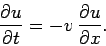(234)

This equation describes the passive advection of some scalar field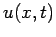carried along by a flow of constant speed. Since the advection equation is somewhat simpler than the wave equation, we shall discuss it first. The advection equation possesses the formal solution(235)

where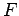is an arbitrary function. This solution describes an arbitrarily shaped pulse which is swept along by the flow, at constant speed, without changing shape.

We seek the solution of Eq. (234) in the region, subject to the simple Dirichlet boundary conditions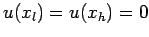. As usual, we discretize in time on the uniform grid, for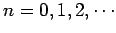. Furthermore, in the-direction, we discretize on the uniform grid, for, where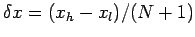. Adopting an explicit temporal differencing scheme, and a centered spatial differencing scheme, Eq. (234) yields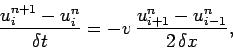(236)

where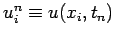. The above equation can be rewritten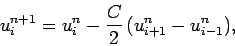(237)

where.

Let us perform a von Neumann stability analysis of the above differencing scheme. Writing, we obtain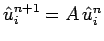, where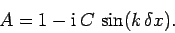(238)

Note that(239)

Thus, the magnitude of the amplification factor is greater than unity for all. This implies, unfortunately, that the simple differencing scheme (237) is unconditionally unstable.Next: The Lax scheme Up: The wave equation Previous: Introduction
Richard Fitzpatrick 2006-03-29